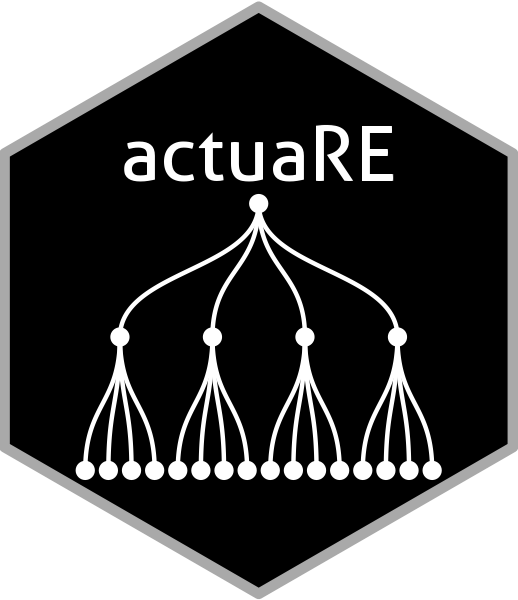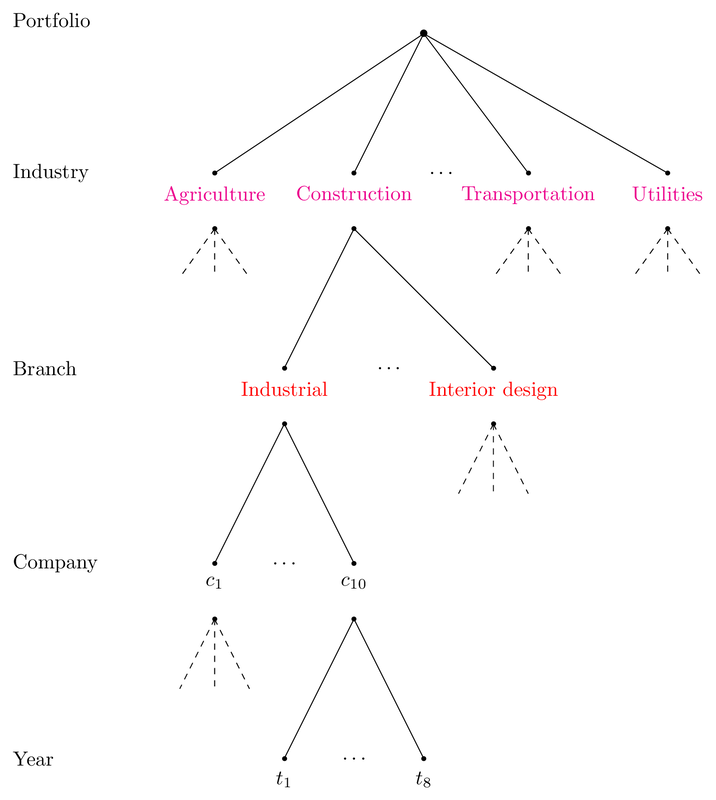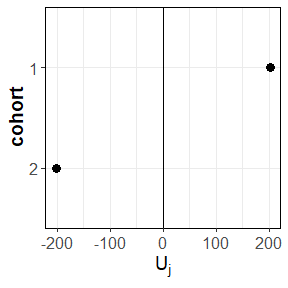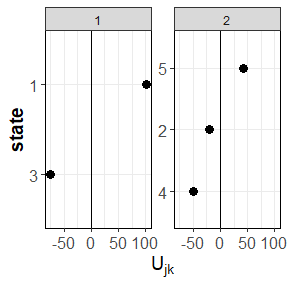# Introduction to actuaRE

#### 2023-03-20In this document, we give you a brief overview of the basic functionality of the actuaRE package. For a more detailed overview of the functions, you can consult the help-pages. Please feel free to send any suggestions and bug reports to the package author.

# Handling hierarchically multi-level factors using random effects models

Multi-level factors (MLFs) are nominal variables with too many levels for ordinary generalized linear model (GLM) estimation (Ohlsson and Johansson 2010). Within the machine learning literature, these type of risk factors are better known as high-cardinality attributes (Micci-Barreca 2001). This package focuses on MLFs that exhibit a hierarchical structure and a typical example hereof, within workers’ compensation insurance, is the NACE code. In our illustration, we work with a hierarchical MLF that has two hierarchical levels: industry and branch. Figure 1 visualizes this hierarchical structure with a hypothetical example.

Figure 1: Hierarchical structure of a hypothetical example## Which random effects models can we fit using actuaRE?

With the current version of the actuaRE package, you are able to fit random effects models with the following functional form

\begin{align*} g(E[Y_{ijkt} | U_j, U_{jk}]) &= \mu + \boldsymbol{x}_{ijkt}^\top \boldsymbol{\beta} + U_j + U_{jk} \\&= \zeta_{ijkt}.\\ \end{align*}

Here, $$Y_{ijkt}$$ denotes the loss cost of risk profile $$i$$ (based on the company-specific risk factors) operating in branch $$k$$ within industry $$j$$ at time $$t$$. We calculate the loss cost as

\begin{align*} Y_{ijkt} = \frac{Z_{ijkt}}{w_{ijkt}} \end{align*} where $$Z_{ijkt}$$ denotes the total claim cost and $$w_{ijkt}$$ is an appropriate volume measure. $$g(\cdot)$$ denotes the link function (for example the identity or log link), $$\mu$$ the intercept, $$\boldsymbol{x}_{ijkt}$$ the company-specific covariate vector and $$\boldsymbol{\beta}$$ the corresponding parameter vector. With the model parameters $$\mu$$ and $$\boldsymbol{\beta}$$ we capture the company-specific effects. To assess the effect of the hierarchical MLF, we introduce the random effects $$U_j$$ and $$U_{jk}$$ which capture the unobservable effects of the industry and the branch in which the company operates. $$U_j$$ denotes the industry-specific deviation from $$\mu + \boldsymbol{x}_{ijkt}^\top \boldsymbol{\beta}$$ and $$U_{jk}$$ denotes the branch-specific deviation from $$\mu + \boldsymbol{x}_{ijkt}^\top \boldsymbol{\beta} + U_{j}$$. We assume that the random industry effects $$U_j$$ are independent and identically distributed (i.i.d.) with $$E[U_j] = 0$$ and $$Var(U_j) = \sigma_{I}^2$$. Similarly, the random branch effects $$U_{jk}$$ are assumed to be i.i.d. with $$E[U_{jk}] = 0$$ and $$Var(U_{jk}) = \sigma_{B}^2$$.

This package offers three different estimation methods to estimate the model parameters:
- Hierarchical credibility model (Jewell 1975)
- Combining the hierarchical credibility model with a GLM (Ohlsson 2008)
- Mixed models (Molenberghs and Verbeke 2005)

### Example data set

To illustrate the functions, we make use of two different data sets. We illustrate the hierarchical credibility model of Jewell (Jewell 1975) using the Hachemeister (Hachemeister 1975) data set. The other functions make use of the dataCar data set.

### Hierarchical credibility model

To estimate the parameters using the hierarchical credibility model, we use the function hierCredibility. By default, the additive hierarchical credibility model (Dannenburg, Kaas, and Goovaerts 1996) is fit

\begin{align*} E[Y_{ijkt} | U_j, U_{jk}] &= \mu + U_j + U_{jk}. \end{align*}

capture.output(library(actuaRE), file = tempfile()) # suppress startup message
#>
#> Package 'actuaRE' version 0.1.5
#>
#> This is version 0.1.5 of actuaRE
data("hachemeisterLong")
fitHC = hierCredibility(ratio, weight, cohort, state, hachemeisterLong)
fitHC
#> Call:
#> hierCredibility(Yijkt = ratio, wijkt = weight, sector = cohort,
#>     group = state, data = hachemeisterLong)
#>
#>
#>
#> Estimated variance parameters:
#>   Individual contracts: 139120026
#>   Var(V[jk]): 11628.45
#>   Var(V[j]): 88476.11
#> Unique number of categories of cohort: 2
#> Unique number of categories of state: 5

To fit the multiplicative hierarchical credibility model (Ohlsson 2005) \begin{align*} E[Y_{ijkt} | \widetilde{U}_j, \widetilde{U}_{jk}] &= \tilde{\mu} \ \widetilde{U}_j \ \widetilde{U}_{jk} \end{align*} you have to specify type = "multiplicative".

fitHCMult = hierCredibility(ratio, weight, cohort, state, hachemeisterLong, type = "multiplicative")
fitHCMult

To get a summary of the model fit, we use the summary function.

summary(fitHC)
#> Call:
#> hierCredibility(Yijkt = ratio, wijkt = weight, sector = cohort,
#>     group = state, data = hachemeisterLong)
#>
#>
#>
#> Estimated variance parameters:
#>   Individual contracts: 139120026
#>   Var(V[jk]): 11628.45
#>   Var(V[j]): 88476.11
#> Unique number of categories of cohort: 2
#> Unique number of categories of state: 5
#>
#> Estimates at the cohort level:
#>
#>    cohort       zj Yjz_BarTilde        qj       Vj        Uj
#> 1:      1 1.427755     1965.436 0.9157058 1946.859  201.8044
#> 2:      2 1.633248     1527.011 0.9255216 1543.250 -201.8044
#>
#> Estimates at the state level:
#>
#>    cohort state    wjk Yjk_BarTilde       zjk      Vjk       Ujk
#> 1:      1     1 100155     2060.921 0.8932938 2048.750 101.89107
#> 2:      1     3  13735     1805.843 0.5344614 1871.491 -75.36785
#> 3:      2     2  19895     1511.224 0.6244749 1523.251 -19.99963
#> 4:      2     4   4152     1352.976 0.2576359 1494.229 -49.02155
#> 5:      2     5  36110     1599.829 0.7511373 1585.748  42.49796

To obtain the fitted values, we use the fitted function

fitted(fitHC)
#>   2048.750 2048.750 2048.750 2048.750 2048.750 2048.750 2048.750 2048.750
#>   2048.750 2048.750 2048.750 2048.750 1871.491 1871.491 1871.491 1871.491
#>  1871.491 1871.491 1871.491 1871.491 1871.491 1871.491 1871.491 1871.491
#>  1523.251 1523.251 1523.251 1523.251 1523.251 1523.251 1523.251 1523.251
#>  1523.251 1523.251 1523.251 1523.251 1494.229 1494.229 1494.229 1494.229
#>  1494.229 1494.229 1494.229 1494.229 1494.229 1494.229 1494.229 1494.229
#>  1585.748 1585.748 1585.748 1585.748 1585.748 1585.748 1585.748 1585.748
#>  1585.748 1585.748 1585.748 1585.748

and we use ranef to extract the estimated random effects.

ranef(fitHC)
#> $sector #> cohort Uj #> 1: 1 201.8044 #> 2: 2 -201.8044 #> #>$group
#>    cohort state       Ujk
#> 1:      1     1 101.89107
#> 2:      1     3 -75.36785
#> 3:      2     2 -19.99963
#> 4:      2     4 -49.02155
#> 5:      2     5  42.49796

We can inspect the estimated random effects using the function plotRE.

ggPlots = plotRE(fitHC, plot = FALSE)
ggPlots[]
ggPlots[]To obtain predictions for a new data frame, we use the predict function.

newDt = hachemeisterLong[sample(1:nrow(hachemeisterLong), 5, F), ]
predict(fitHC, newDt)
#>  2048.750 1523.251 1523.251 1585.748 1585.748

### Combining the hierarchical credibility model with a GLM

To allow for company-specific risk factors, we extend the multiplicative hierarchical credibility model to \begin{align*} E[Y_{ijkt} | \widetilde{U}_j, \widetilde{U}_{jk}] &= \tilde{\mu} \ \gamma_{ijkt} \ \widetilde{U}_j \ \widetilde{U}_{jk} = \gamma_{ijkt} V_{jk} \end{align*} where $$\gamma_{ijkt}$$ denotes the effect of the company-specific covariates. To estimate this model using Ohlsson’s GLMC algorithm (Ohlsson 2008), we use can either use the function hierCredGLM or hierCredTweedie. hierCredGLM allows the user to specify the power parameter $$p$$. Conversely, hierCredTweedie estimates the power parameter $$p$$ along with the other parameters using the cpglm function from the cplm package.

data("dataCar")
fit = hierCredGLM(Y ~ area + (1 | VehicleType / VehicleBody), dataCar, weights = w)
summary(fit)
#> Call:
#> hierCredGLM(formula = Y ~ area + (1 | VehicleType/VehicleBody),
#>     data = dataCar, weights = w)
#>
#>
#> Combination of the hierarchical credibility model with a GLM
#>
#> Estimated variance parameters:
#>   Individual contracts: 4288715
#>   Var(V[jk]): 349.22
#>   Var(V[j]): 628.379
#> Unique number of categories of VehicleType: 2
#> Unique number of categories of VehicleBody: 9
#>
#> Results contract-specific risk factors:
#>
#>
#> Call:
#> glm(formula = FormulaGLM, family = tweedie(var.power = p, link.power = 0),
#>     data = data, weights = data$wijkt, model = T, y = T) #> #> Deviance Residuals: #> Min 1Q Median 3Q Max #> -9.321 -6.751 -5.171 -3.311 111.342 #> #> Coefficients: #> Estimate Std. Error t value Pr(>|t|) #> (Intercept) 5.63582 0.08857 63.630 <2e-16 *** #> areaB 0.04804 0.13070 0.368 0.7132 #> areaC 0.07686 0.11758 0.654 0.5133 #> areaD -0.18602 0.15779 -1.179 0.2384 #> areaE 0.13070 0.16694 0.783 0.4337 #> areaF 0.46429 0.18616 2.494 0.0126 * #> --- #> Signif. codes: 0 '***' 0.001 '**' 0.01 '*' 0.05 '.' 0.1 ' ' 1 #> #> (Dispersion parameter for Tweedie family taken to be 978.1447) #> #> Null deviance: 3308780 on 67565 degrees of freedom #> Residual deviance: 3298572 on 67560 degrees of freedom #> AIC: NA #> #> Number of Fisher Scoring iterations: 7 We use the same syntax as used by the package lme4 to specify the model formula. Here, (1 | VehicleType / VehicleBody) specifies a random effect $$U_j$$ for VehicleType and a nested random effect $$U_{jk}$$ for VehicleBody. We extract the estimated parameters using fixef (company-specific effects) and ranef (random effects). fixef(fit) #> (Intercept) areaB areaC areaD areaE areaF #> 5.63581573 0.04803789 0.07686029 -0.18601585 0.13069527 0.46428870 ranef(fit) #>$sector
#>         VehicleType        Uj
#> 1:   Common vehicle 0.9563139
#> 2: Uncommon vehicle 1.0514319
#>
#> group #> VehicleType VehicleBody Ujk #> 1: Common vehicle HBACK 1.0249732 #> 2: Common vehicle SEDAN 0.9526347 #> 3: Common vehicle UTE 0.9970046 #> 4: Uncommon vehicle COUPE 1.0232527 #> 5: Uncommon vehicle HDTOP 1.0064355 #> 6: Uncommon vehicle MIBUS 1.0041799 #> 7: Uncommon vehicle PANVN 1.0023171 #> 8: Uncommon vehicle STNWG 0.9833459 #> 9: Uncommon vehicle TRUCK 1.0076538 In addition, the same functions as before can be used. head(fitted(fit)) #> 1 2 3 4 5 6 #> 296.6857 274.7367 304.5521 240.6055 296.6857 320.2954 predict(fit, newdata = dataCar[1:2, ], type = "response") #> 1 2 #> 296.6857 274.7367 ggPlots = plotRE(fit, plot = FALSE) ### Mixed models Alternatively, we can rely on the mixed models framework (Molenberghs and Verbeke 2005) to estimate the model parameters. Here, we can use the function to estimate a Tweedie generalized linear mixed model. Fitting the model, however, takes quite some time. We can speed up the fitting process by providing some initial estimates and this is exactly what the tweedieGLMM function does! Nonetheless, even with the initial estimates the fitting process does take some time (approximately 5 minutes using Windows 10 with an intel i7 and 32 gigabytes of RAM). fitGLMM = tweedieGLMM(Y ~ area + (1 | VehicleType / VehicleBody), dataCar, weights = w, verbose = TRUE) ### Balance property For insurance applications, it is crucial that the models provide us a reasonable premium volume at portfolio level. Hereto, we examine the balance property [Bühlmann and Gisler (2006)](Wüthrich 2020) on the training set. That is, \begin{equation} \begin{aligned} \sum_{i, j, k, t} w_{ijkt} \ Y_{ijkt} &= \sum_{i, j, k, t} w_{ijkt} \ \widehat{Y}_{ijkt}\\ \end{aligned} \end{equation} where $$i$$ serves as an index for the tariff class. GLMs fulfill the balance property when we use the canonical link (see (Wüthrich 2020)). For LMMs and hence, the hierarchical credibility model this property also holds. Conversely, most GLMMs do not have this property. To regain the balance property, we introduce a quantity $$\alpha$$ \begin{equation} \begin{aligned} \alpha &= \frac{\sum_{i, j, k, t} w_{ijkt} \ Y_{ijkt}}{\sum_{i, j, k, t} w_{ijkt} \ \widehat{Y}_{ijkt}}\\ \end{aligned} \end{equation} which quantifies the deviation of the total predicted damage from the total observed damage. In case of the log link, we can then use $$\alpha$$ to update the intercept to $$\hat{\mu} + \log(\alpha)$$ to regain the balance property. By default, the intercept is updated when fitting models using hierCredGLM, hierCredTweedie and tweedieGLMM. If you do not wish to update the intercept, you can set the argument balanceProperty = FALSE. fitnoBP = hierCredGLM(Y ~ area + (1 | VehicleType / VehicleBody), dataCar, weights = w, balanceProperty = F) yHatnoBP = fitted(fitnoBP) w = weights(fitnoBP, "prior") y = fitnoBPy

fitBP  = hierCredGLM(Y ~ area + (1 | VehicleType / VehicleBody), dataCar, weights = w, balanceProperty = T)
yHatBP = fitted(fitBP)

sum(w * y) / sum(w * yHatnoBP)
#>  1.001388
sum(w * y) / sum(w * yHatBP)
#>  1

Alternatively, you can use the build-in function BalanceProperty. You can use this function with any object that has the slots fitted, weights and y.

BalanceProperty(fitnoBP)
#> Warning in BalanceProperty(fitnoBP):
#> Balance property is not satisfied.
#>
#> Ratio total observed damage to total predicted damage: 1.001388
BalanceProperty(fitBP)
#>
#> Balance property is satisfied.